Next: Vector Product Up: Vectors and Vector Fields Previous: Scalar Product

# Vector Area

Suppose that we have planar surface of scalar area. We can define a vector area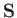whose magnitude is, and whose direction is perpendicular to the plane, in the sense determined by a right-hand circulation rule (see Section A.8) applied to the rim, assuming that a direction of circulation around the rim is specified. (See Figure A.7.) This quantity clearly possesses both magnitude and direction. But is it a true vector? We know that if the normal to the surface makes an anglewith the-axis then the area seen looking along the-direction is. This is the-component of(because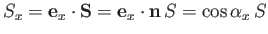, where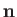is the unit normal to the surface). Similarly, if the normal makes an angle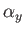with the-axis then the area seen looking along the-direction is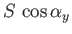. This is the-component of. If we limit ourselves to a surface whose normal is perpendicular to the-direction then. It follows that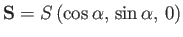. If we rotate the basis about the-axis by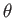degrees, which is equivalent to rotating the normal to the surface about the-axis by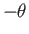degrees, so that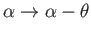, then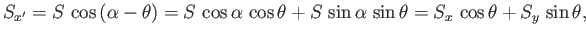(A.33)

which is the correct transformation rule for the-component of a vector. The other components transform correctly as well. This proves both that a vector area is a true vector, and that the components of a vector area are the projected areas seen looking down the coordinate axes.According to the vector addition theorem, the projected area of two plane surfaces, joined together at a line, looking along the-direction (say) is the-component of the resultant of the vector areas of the two surfaces. Likewise, for many joined-up plane areas, the net area seen looking down the-axis, which is the same as the area of the outer rim seen looking down the-axis, is the-component of the resultant of all the vector areas: that is,(A.34)

If we approach a limit, by letting the number of plane facets increase, and their areas reduce, then we obtain a continuous surface denoted by the resultant vector area(A.35)

It is clear that the area of the rim seen looking down the-axis is just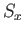. Similarly, for the areas of the rim seen looking down the other coordinate axes. Note that it is the rim of the surface that determines the vector area, rather than the nature of the surface spanning the rim. So, two different surfaces sharing the same rim both possess the same vector area.

In conclusion, a loop (not all in one plane) has a vector areawhich is the resultant of the component vector areas of any surface ending on the loop. The components ofare the areas of the loop seen looking down the coordinate axes. As a corollary, a closed surface has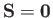, because it does not possess a rim.Next: Vector Product Up: Vectors and Vector Fields Previous: Scalar Product
Richard Fitzpatrick 2016-03-31